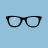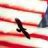# How to convert Boolean to integer?

D

#### dodoei

##### New member
If I have def N=A>B+B>C+C>D how do I convert the result to 3, that is if all three comparisons are true, N will be 3? Thanks!

T

#### tomsk

##### Well-known member
VIP
Warehouse
Define three conditions and them sum them. If all true, then the value 3 will be returned.

def cond1 = A > B;
def cond2 = B > C;
def cond3 = C > D;

def result = cond1 + cond2 + cond3;

D

#### dodoei

##### New member
Define three conditions and them sum them. If all true, then the value 3 will be returned.

def cond1 = A > B;
def cond2 = B > C;
def cond3 = C > D;

def result = cond1 + cond2 + cond3;
Thanks a lot! What I plan to do is to rank 4 to 5 variables, there seems no easy way or function to do it except to compare them one by one. So in this case I guess I would need to def all 30 cond? Thanks again!

T

#### tomsk

##### Well-known member
VIP
Warehouse
Thanks a lot! What I plan to do is to rank 4 to 5 variables, there seems no easy way or function to do it except to compare them one by one. So in this case I guess I would need to def all 30 cond? Thanks again!
I have in the past been asked how to compare the lowest and highest of 3 variables.
Here's one way to do so

def lowest = min(a, min(b,c));
def highest = max(a, max(b,c));

So with 5 variables in your case you can adopt a similar nested approach although that would get rather complex pretty quick

D

#### dodoei

##### New member
By the way, I found in addchartbubble, I can not use the current value for the price location, for example, Data, I have to use Data
I have in the past been asked how to compare the lowest and highest of 3 variables.
Here's one way to do so

def lowest = min(a, min(b,c));
def highest = max(a, max(b,c));

So with 5 variables in your case you can adopt a similar nested approach although that would get rather complex pretty quick
Thanks!Questions 12Questions 10